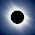# How to convert Hexadecimal to Decimal, Binary and Octal in Java program - Example

Hexadecimal to decimal and binary conversion in Java
This Java program will convert Hexadecimal number to decimal, binary and Octal in Java programming language using JDK standard API methods. For beginners hexadecimal is base 16 number, while decimal is base 10, Octal is base 8 and binary is base 2 numbers in Number systems. Binary only contains 0 and 1 bits, octal contains 0 to 7, decimal contains 0 to 9 and Hexadecimal contains 0-9,A,B,C,D,E,F where F represent 16. Thankfully Java library provides convenient method to convert any integer from one number system to another. In our last article we have seen How to convert decimal number to binary in Java and in this article we will convert Hexadecimal numbers to binary, octal and decimal numbers.If you are going for programming interviews, then its better to prepare How to do this exercise without using Java API as well, as interviewer commonly expect programmer to create there own method during interviews e.g. reversing String without using StringBuffer reverse() method.

## Hexadecimal to Decimal, binary and Octal in JavaJava API provides two methods which is used in converting number from one number system to other. One is Integer.parseInt() which is used to convert String to Integer in Java but also allows you to specify radix or base. Which means by using parseInt() you can convert any Hexadecimal String to decimal number in Java. Same technique can be used to convert binary String or Octal String to decimal number in Java. Once you got an Integer object representing Decimal number you can use Integer.toBinaryString() and Integer.toOctalString() to convert same Hexadecimal number into Binary and Octal in Java.

here is complete code example of converting Hexadecimal number to Binary, decimal and Octal in Java.

/**
*
* Java program to convert Hexadecimal to binary, decimal and Octal in Java.
* Hexadecimal is base 16, Decimal number is base 10, Octal is base 8
* and Binary is base 2 number which has just two numbers 0 and 1.
* @author
*/

public class ConvertHexaToDecimal {

public static void main(String args[]) {
// Ask user to enter an Hexadecimal number in Console
Scanner scanner = new Scanner(System.in);

String hexadecimal = scanner.next();

//Converting Hexa decimal number to Decimal in Java
int decimal = Integer.parseInt(hexadecimal, 16);

System.out.println("Converted Decimal number is : " + decimal);

//Converting hexa decimal number to binary in Java
String binary = Integer.toBinaryString(decimal);
System.out.printf("Hexadecimal to Binary conversion of %s is %s %n", hexadecimal, binary );

// Converting Hex String to Octal in Java
String octal = Integer.toOctalString(decimal);
System.out.printf("Hexadecimal to Octal conversion of %s is %s %n", hexadecimal, octal );
}
}

Output:
A
Converted Decimal number is : 10
Hexadecimal to Binary conversion of A is 1010
Hexadecimal to Octal conversion of A is 12

An advantage of using Java API for Hexadecimal to Decimal, Binary and Octal conversion is that, Above code can also convert negative Hexadecimal number to binary, octal and decimal in Java as shown in below Output:

-B
Converted Decimal number is : -11
Hexadecimal to Binary conversion of -B is 11111111111111111111111111110101
Hexadecimal to Octal conversion of -B is 37777777765

That's all on How to convert Hexadecimal number to Decimal in Java. By using similar technique we can also convert Hex numbers to binary and Octal as well. Let us know if you come across any other way to convert hex number to binary, decimal and Octal in Java.

Further Learning
Data Structures and Algorithms: Deep Dive Using Java
Java Fundamentals: The Java Language
Complete Java Masterclass

Some other Conversion tutorial from Java

1.A very good tutorial for beginner like me. Thanks a lot !

2.great simple and easy thx

3.Thank you so much, I've been struggling with this for quite a while. :)

1.Hello @Annoymous, glad that you find this code of hexadecimal to binary and decimal conversion useful.

4.